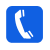020-32218669## 各种表面活性剂性能一览表

 表面活性剂名称 40度 70度 100度 AEO-5 片碱15 g/L 片碱13 g/L 片碱 3g/L AEO-7 片碱22 g/L 片碱14 g/L 片碱 5g/L AEO-9 片碱30 g/L 片碱24 g/L 片碱 12g/L TX-10 片碱19 g/L 片碱15 g/L 片碱 6g/L OP-10 片碱27 g/L 片碱22 g/L 片碱11 g/L 渗透剂JFC 片碱21 g/L 片碱16 g/L 片碱 9g/L 快T 片碱10 g/L 片碱7 g/L 片碱 3g/L 净洗剂209 片碱18 g/L 片碱13 g/L 片碱 5g/L EL-80 片碱29 g/L 片碱22 g/L 片碱8 g/L 吐温80 片碱22 g/L 片碱11 g/L 片碱7 g/L 斯潘80 片碱14 g/L 片碱13 g/L 片碱5 g/L 十二烷基苯磺酸钠 LAS 片碱24 g/L 片碱16 g/L 片碱 9g/L 十二烷基硫酸钠 SDS 片碱81 g/L 片碱44 g/L 片碱 15g/L 仲烷基磺酸钠SAS 片碱30 g/L 片碱22 g/L 片碱 12g/L 烯基磺酸钠-AOS 片碱29 g/L 片碱20 g/L 片碱13 g/L 椰油脂肪酸二乙醇酰胺 片碱18 g/L 片碱8 g/L 片碱3 g/L 脂肪醇醚硫酸盐 AES 片碱98 g/L 片碱77 g/L 片碱 35g/L 脂肪醇醚羧酸盐 AEC 片碱111 g/L 片碱79 g/L 片碱 40g/L 脂肪酸甲酯磺酸盐MES （液体） 片碱145 g/L 片碱95 g/L 片碱 60g/L 脂肪醇的磷酸盐 片碱180 g/L 片碱135 g/L 片碱 110g/L 脂肪醇醚的磷酸酯 片碱210 g/L 片碱147 g/L 片碱 170g/L

R()=F2-F1

R值越大，表明净洗能力越强，该测试标准可用来表征表面活性剂对一般污垢的去除，不适用于反映油脂和蜡质的去除能力。

 表面活性剂名称 R（%）值 AEO-3 R（%）=3.69 AEO-5 R（%）=3.31 AEO-7 R（%）=9.50 AEO-9 R（%）=12.19 TX-10 R（%）=15.77 NP-8.6 R（%）=14.98 OP-10 R（%）=14.55 XL-90 R（%）=13.91 XP-90 R（%）=4.30 TO-90 R（%）=15.58 渗透剂JFC R（%）=2.01 快T R（%）=0.77 净洗剂209 R（%）=4.98 十二烷基苯磺酸钠 LAS R（%）=9.12 十二烷基硫酸钠 SDS R（%）=5.30 烯基磺酸钠-AOS R（%）=8.63 仲烷基磺酸钠SAS R（%）=15.81 脂肪醇醚硫酸盐 AES R（%）=5.91 脂肪醇醚羧酸盐 AEC R（%）=6.20 脂肪酸甲酯磺酸盐MES （液体） R（%）=15.55 脂肪醇的磷酸盐 R（%）=2.08 脂肪醇醚的磷酸酯AEP R（%）=5.88

C=试样去油质量／标准配方去油质量，C值越大，表明表面活性剂的去油能力越强

 表面活性剂名称 去油C值 AEO-3 去油C值=1.53 AEO-5 去油C值=1.40 AEO-7 去油C值=1.22 AEO-9 去油C值=1.01 TX-10 去油C值=1.17 NP-8.6 去油C值=1.25 OP-10 去油C值=1.37 XL-90 去油C值=1.10 XP-90 去油C值=0.66 TO-90 去油C值=1.40 渗透剂JFC 去油C值=0.77 脂肪酸甲酯乙氧基化物FMEE 去油C值=1.94 快T 去油C值=0.35 净洗剂209 去油C值=0.76 十二烷基苯磺酸钠 LAS 去油C值=0.92 十二烷基硫酸钠 SDS 去油C值=0.81 烯基磺酸钠-AOS 去油C值=0.73 仲烷基磺酸钠SAS 去油C值=0.98 脂肪醇醚硫酸盐 AES 去油C值=0.63 脂肪醇醚羧酸盐 AEC 去油C值=0.72 脂肪酸甲酯磺酸盐MES （液体） 去油C值=1.11 脂肪醇的磷酸盐 去油C值=0.32 脂肪醇醚的磷酸酯AEP 去油C值=0.46

1， 标准蜡布的制备

2 测试方法

 表面活性剂名称 W值 AEO-3 W=67.42 AEO-5 W=61.98 AEO-7 W=53.25 AEO-9 W=47.30 TX-10 W=46.11 NP-8.6 W=60.03 OP-10 W=58.92 XL-90 W=48.54 XP-90 W=33.16 TO-7 W=68.96 TO-9 W=59.81 脂肪酸甲酯乙氧基化物FMEE W=77.43 三乙醇胺 W=49.79 三乙醇胺油酸皂 W=56.31 净洗剂6501 W=32.78 渗透剂JFC W=31.91 快T W=18.90 净洗剂209 W=22.55 十二烷基苯磺酸钠 LAS W=34.17 十二烷基硫酸钠 SDS W=27.31 烯基磺酸钠-AOS W=29.25 仲烷基磺酸钠SAS W=30.87 脂肪醇醚硫酸盐 AES W=26.37 脂肪醇醚羧酸盐 AEC W=33.88 脂肪酸甲酯磺酸盐MES （液体） W=49.35 脂肪醇的磷酸盐 W=20.47 脂肪醇醚的磷酸酯AEP W=29.38

【推荐阅读】